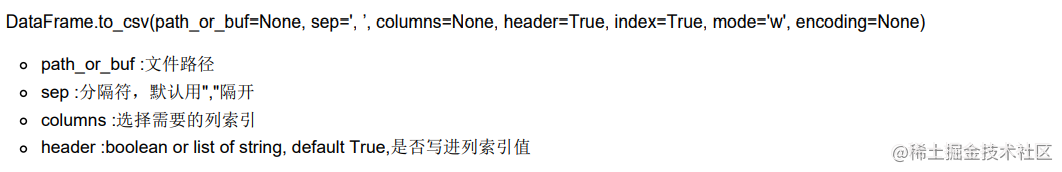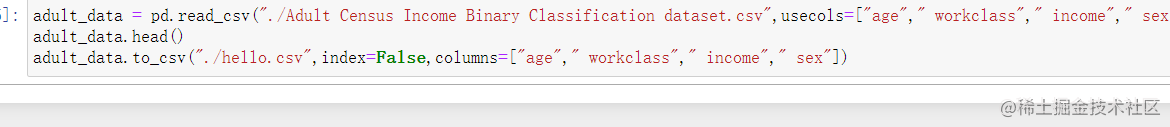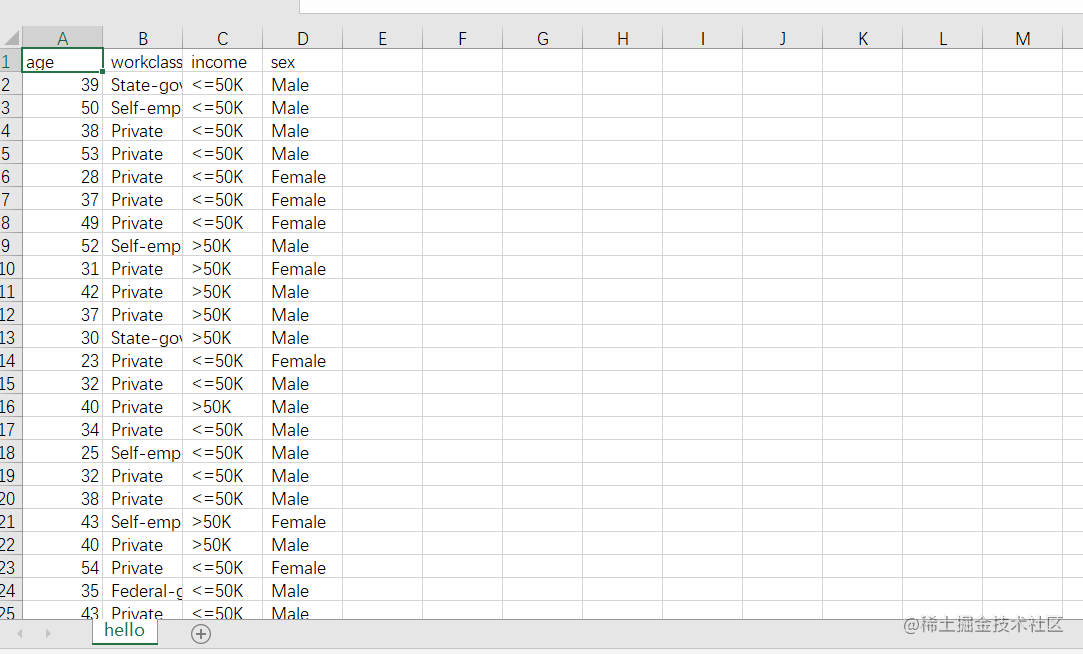# Pandas基礎使用（機器學習基礎）

theme: channing-cyan highlight: agate

「這是我參與2022首次更文挑戰的第5天，活動詳情檢視：2022首次更文挑戰

# 前言

import pymysql import pandas as pd

# 儲存

data_sql.to_csv("test.csv") ```

con = create_engine('mysql+pymysql://user_name:[email protected]:3306/database_name') data = pd.read_sql_table("table_name", con) data.to_csv("table_name.csv") ``` 所以說，理論上如果你只是想要玩資料操作的話，你甚至可以不需要專門去學習sql（當然最好還是學習的，但如果你是辦公人群，只是有特殊需求的話，確實可以考慮）

# Pandas的基本資料結構

## Series線性結構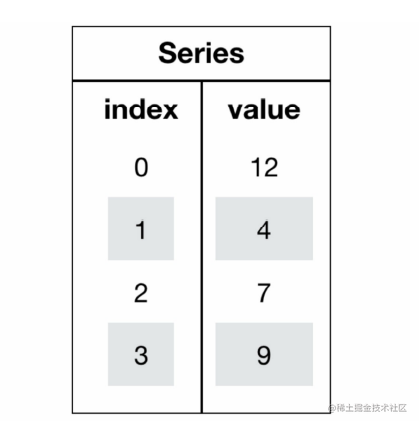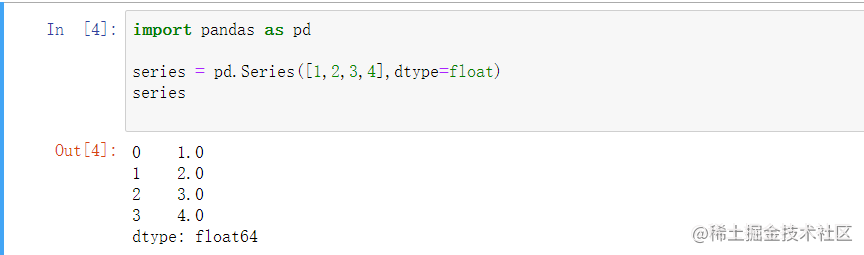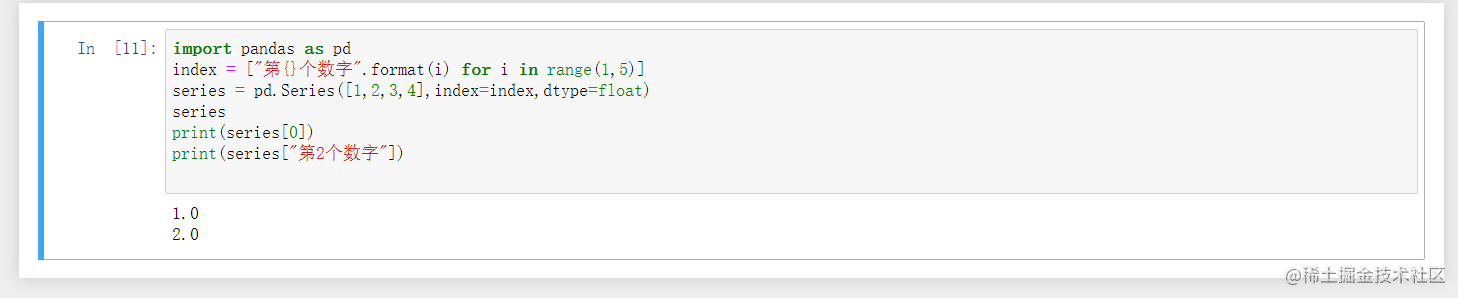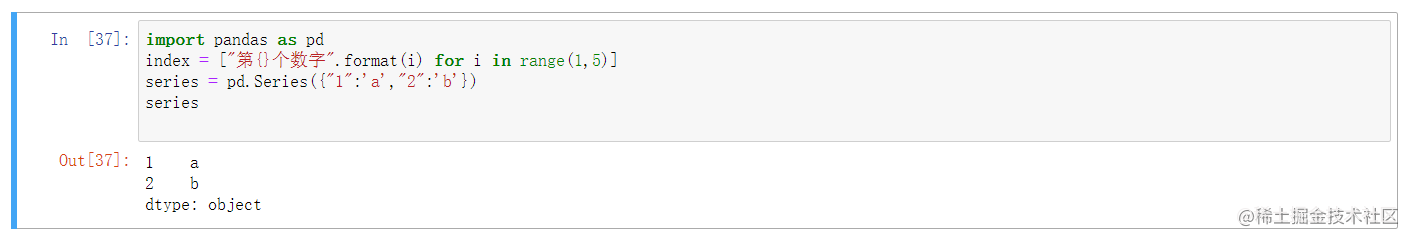```python import pandas as pd index = ["第{}個數字".format(i) for i in range(1,5)] series = pd.Series([1,2,3,4],index=index,dtype=float) series print(series) print(series["第2個數字"]) print(series[1:3])

```

```python import pandas as pd index = ["第{}個數字".format(i) for i in range(1,5)] series = pd.Series([1,2,3,4],index=index,dtype=float) series

# print(series[1:3])

index_new = ["第{}個數字New".format(i) for i in range(1,5)] series.index = index_new series.reset_index(drop=True) series ```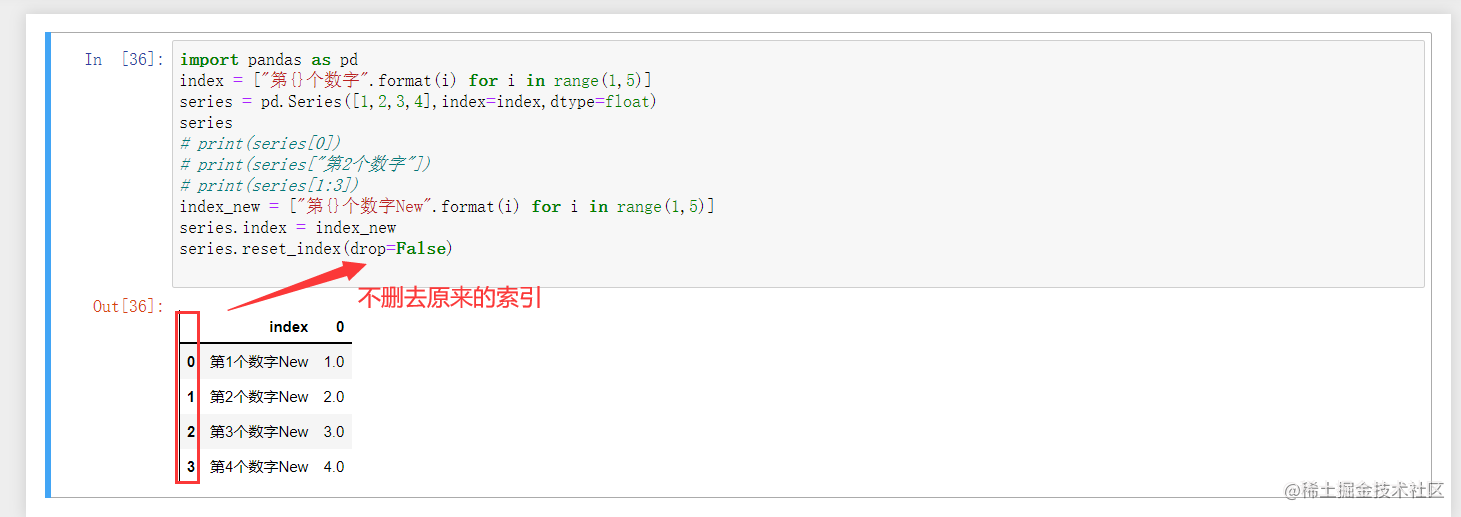## DataFrame結構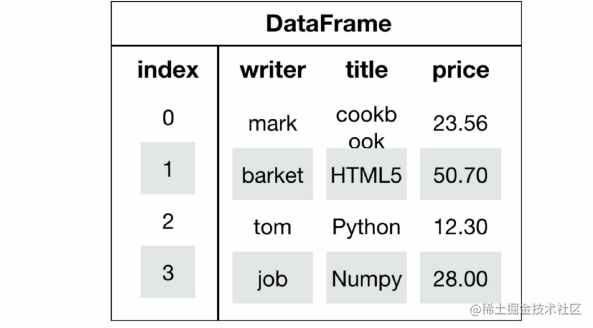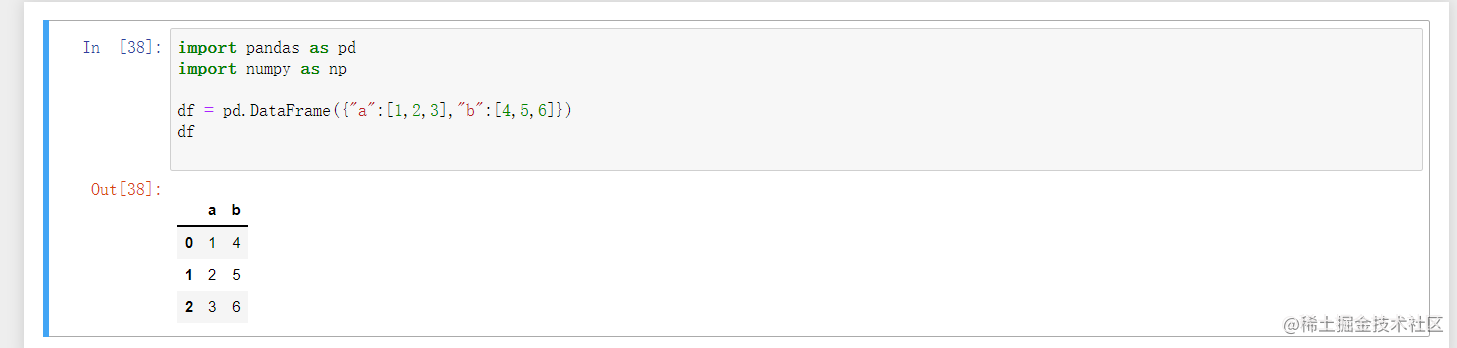```python import pandas as pd import numpy as np

score = np.random.randint(60, 100, (10, 5))

# 構造行索引序列

subjects = ["語文", "數學", "英語", "政治", "體育"]

# 構造列索引序列

stu = ['同學' + str(i) for i in range(1,score.shape+1)]

df = pd.DataFrame(score,columns=subjects,index=stu) df ```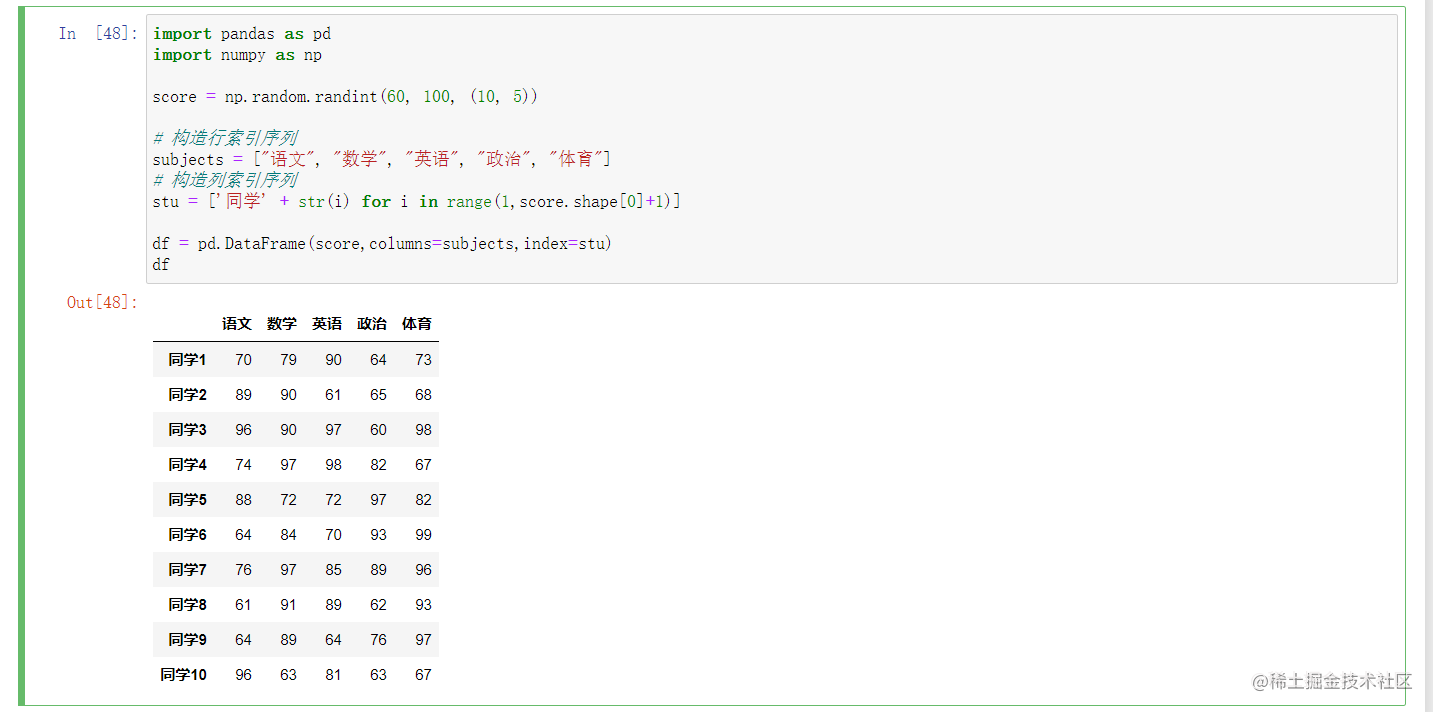# 對DataFrame進行操作

## 切片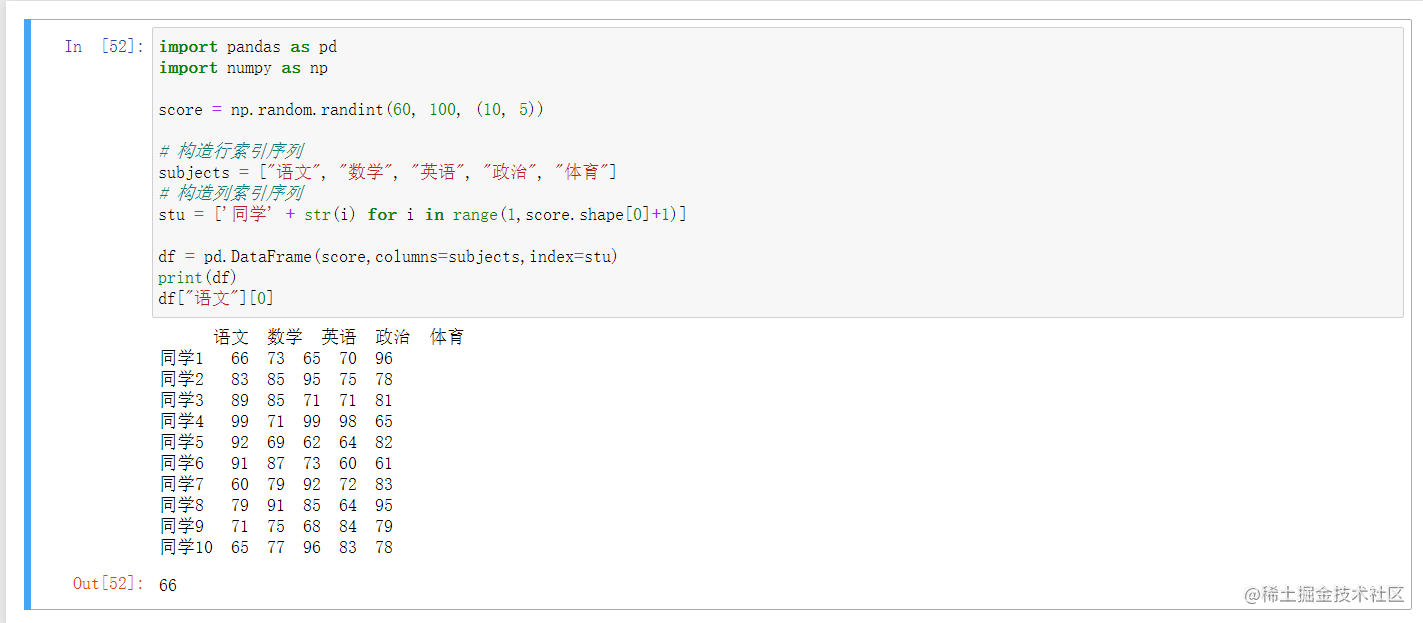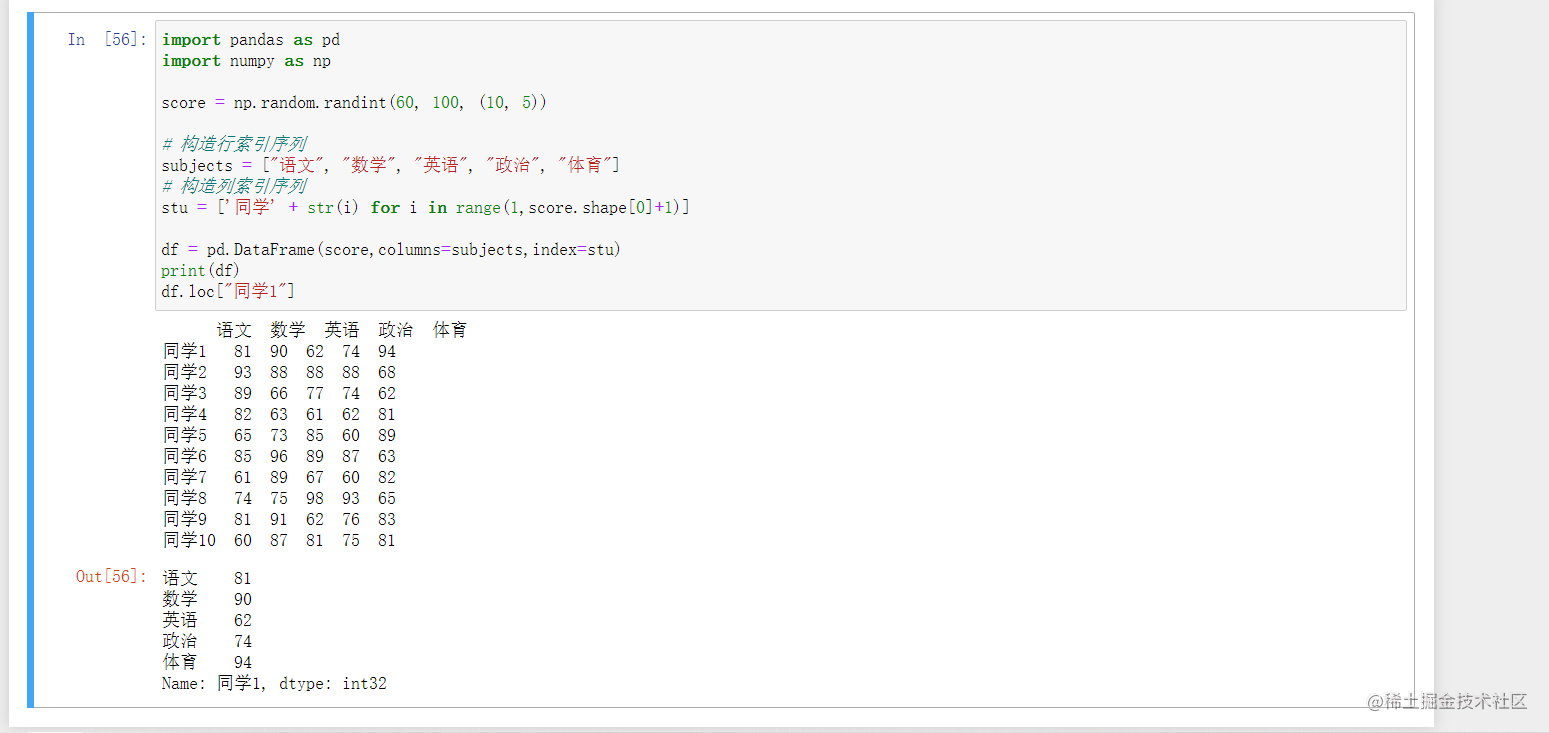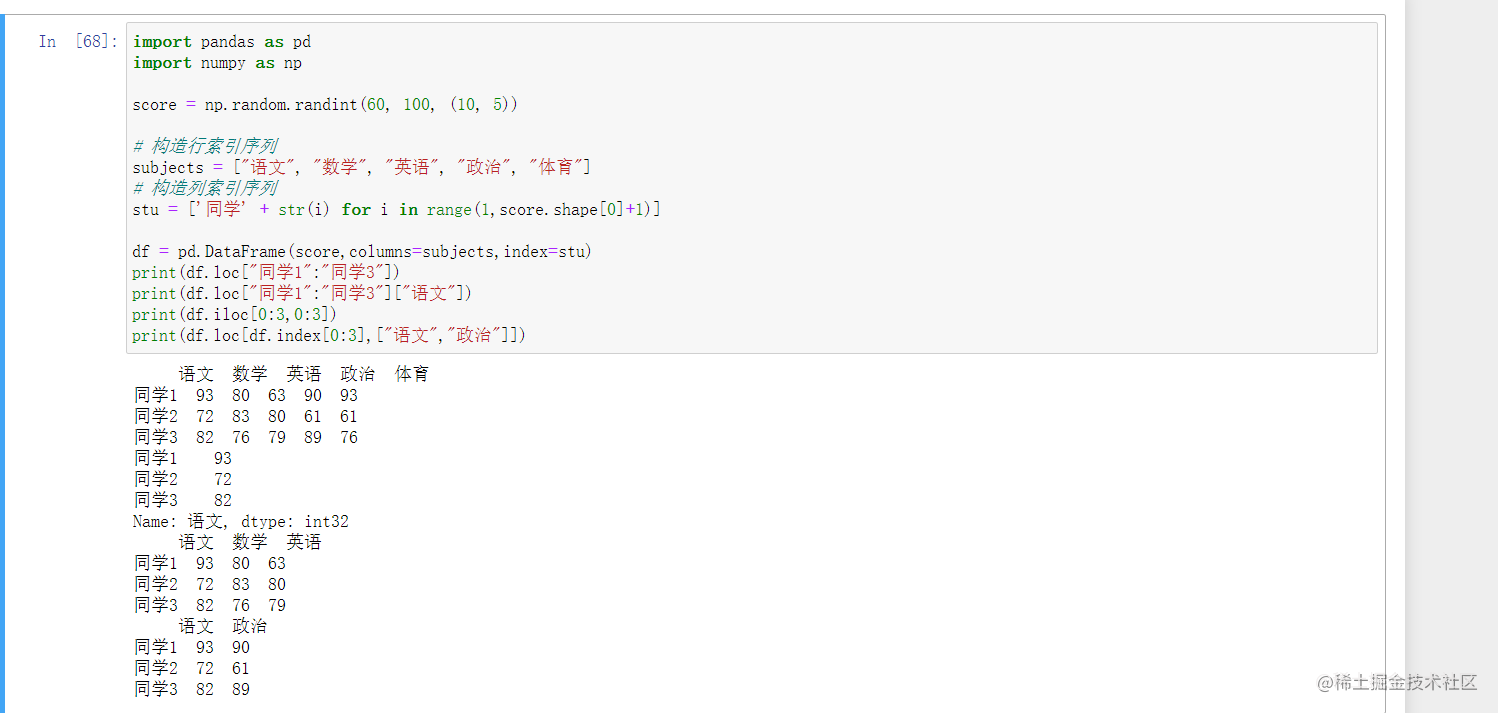```python import pandas as pd import numpy as np

score = np.random.randint(60, 100, (10, 5))

# 構造行索引序列

subjects = ["語文", "數學", "英語", "政治", "體育"]

# 構造列索引序列

stu = ['同學' + str(i) for i in range(1,score.shape+1)]

df = pd.DataFrame(score,columns=subjects,index=stu) print(df.loc["同學1":"同學3"]) print(df.loc["同學1":"同學3"]["語文"]) print(df.iloc[0:3,0:3]) print(df.loc[df.index[0:3],["語文","政治"]]) ```

## 轉置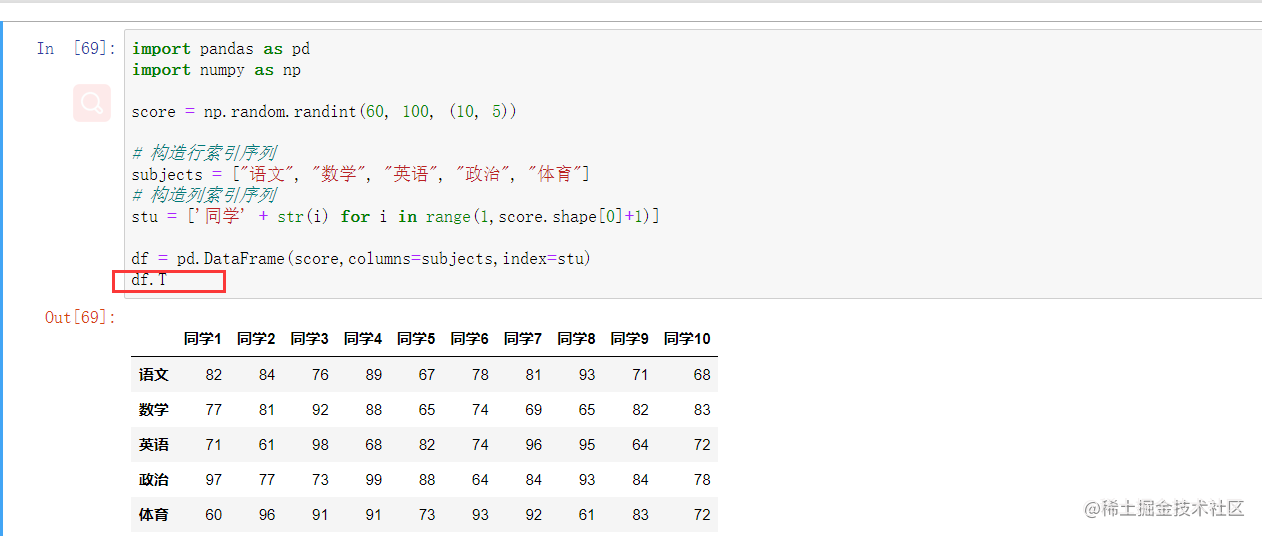## 排序

### 對DataFrame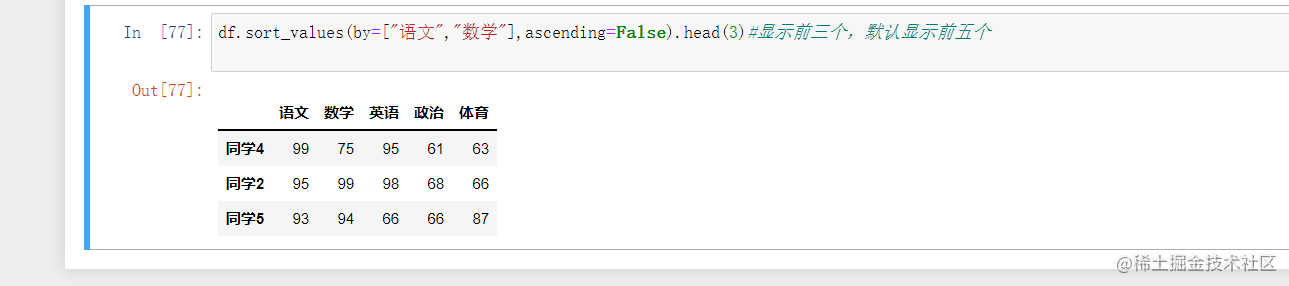### 對Series排序

`df["語文"].sort_values(ascending=False)`

# 這裡注意的是改變之後我們的df需要重新接受

print(df) ```

### 修改索引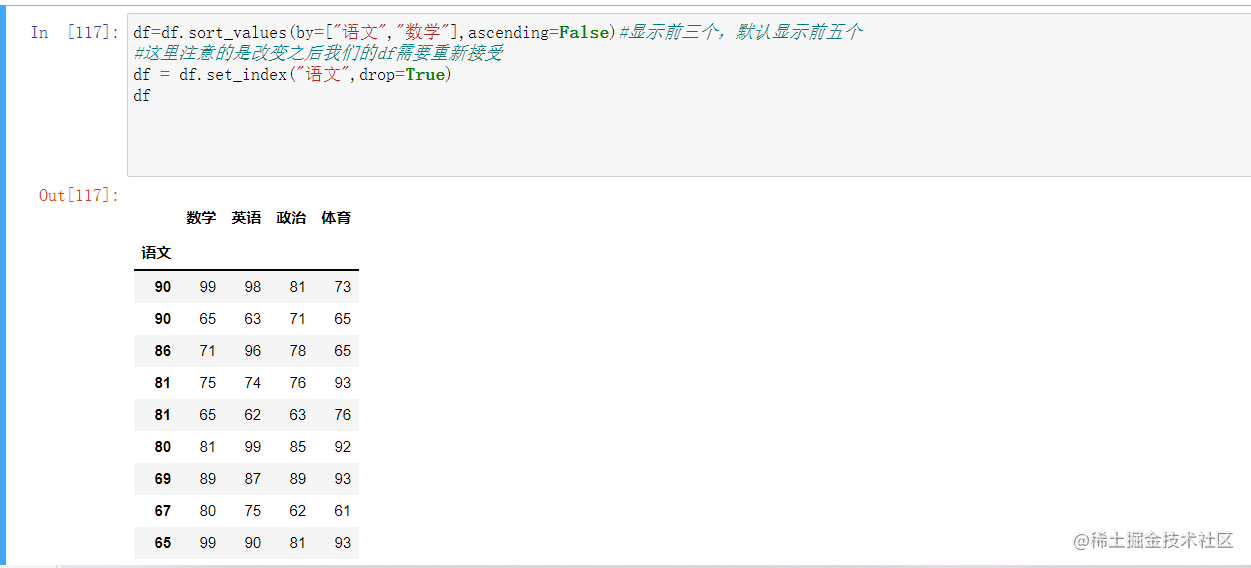注意我們這裡的話其實也是可以設定多個索引的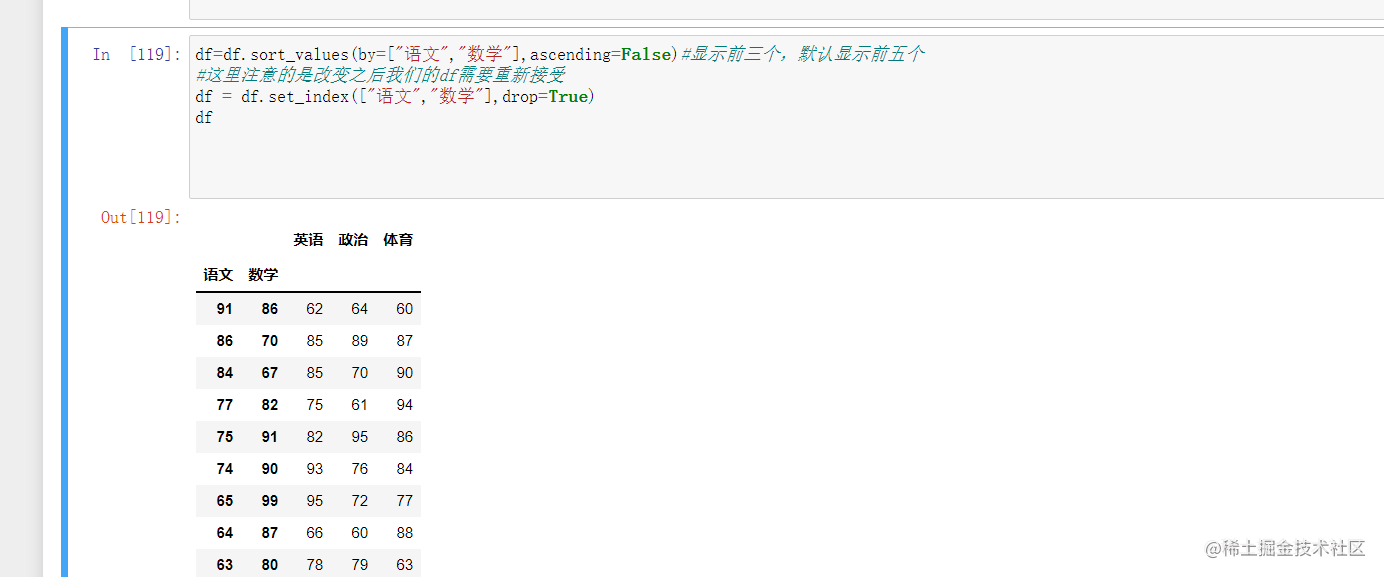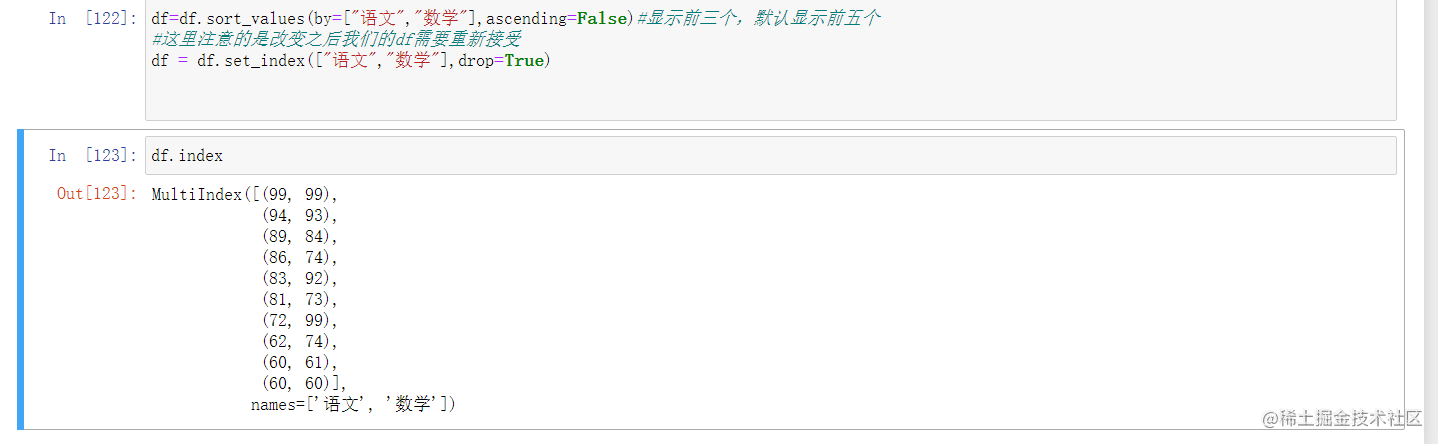## 運算

### 邏輯運算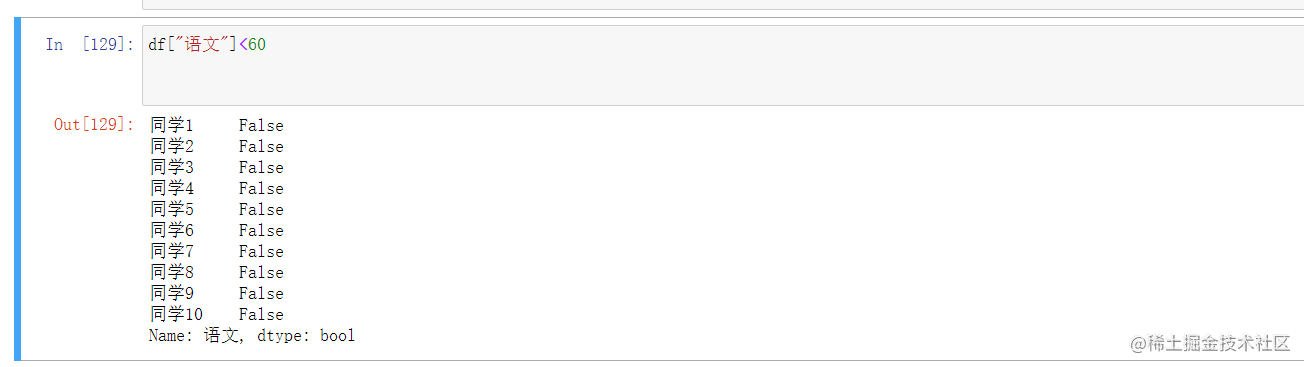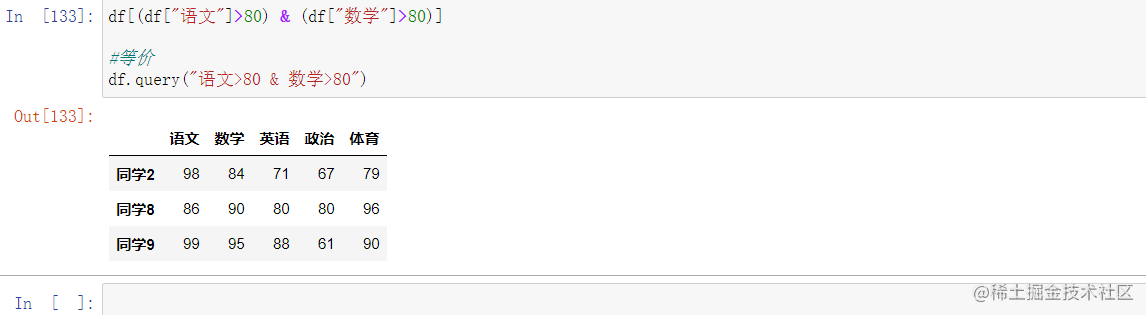### 統計運算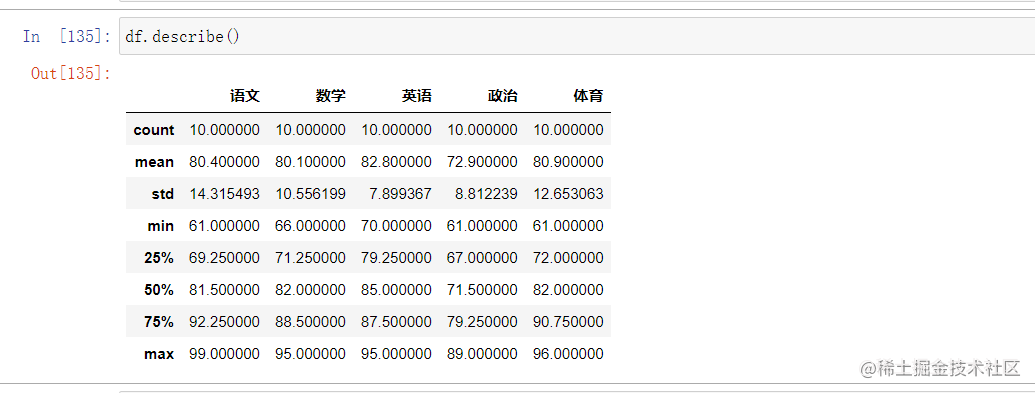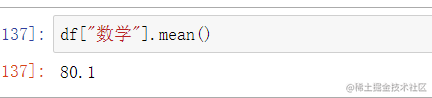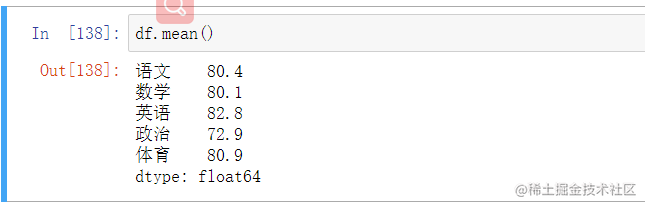• min(最小值)
• max(最大值)
• mean(平均值)
• median(中位數)
• var(方差)
• std(標準差)
• mode(眾數)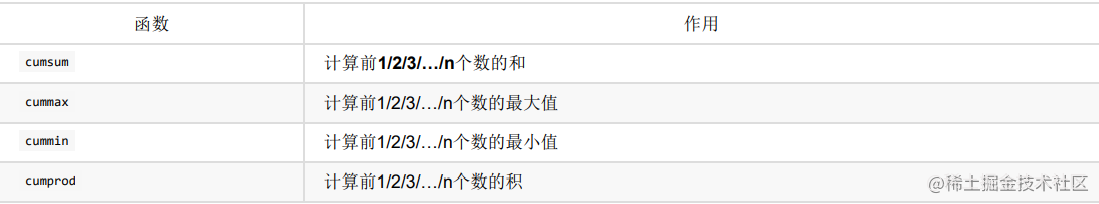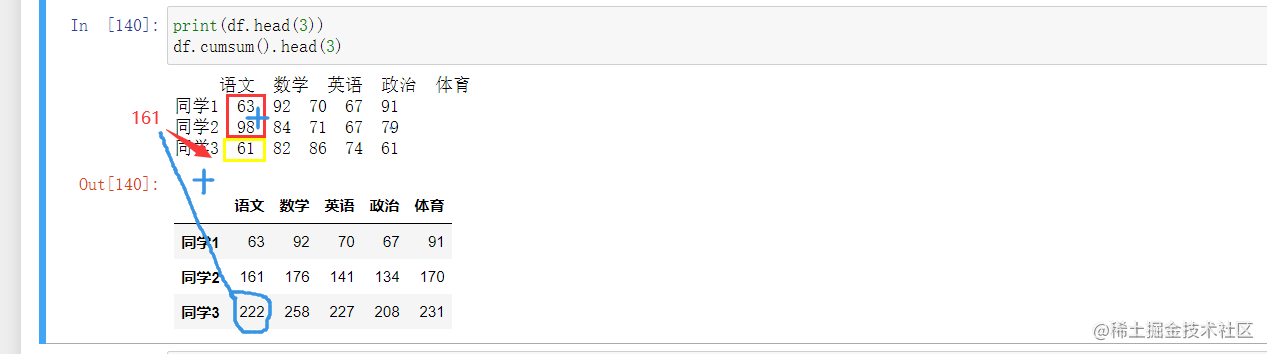### 自定義運算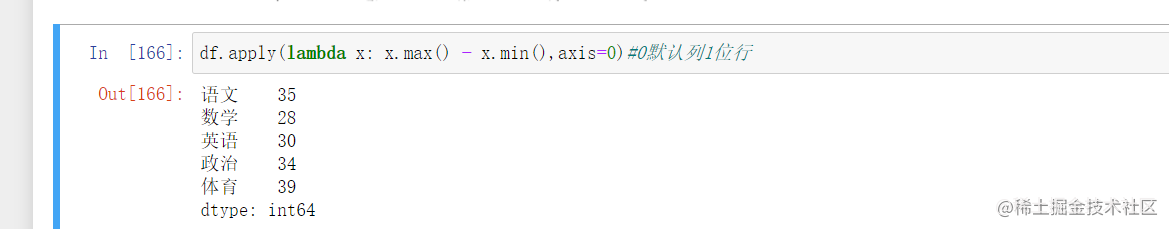## 繪圖

```python import matplotlib.pyplot as plt from pylab import mpl

# 設定顯示中文字型

mpl.rcParams["font.sans-serif"] = ["SimHei"]

# 設定正常顯示符號

mpl.rcParams["axes.unicode_minus"] = False tx1 = df.loc["同學1"] tx1.plot() plt.show()

```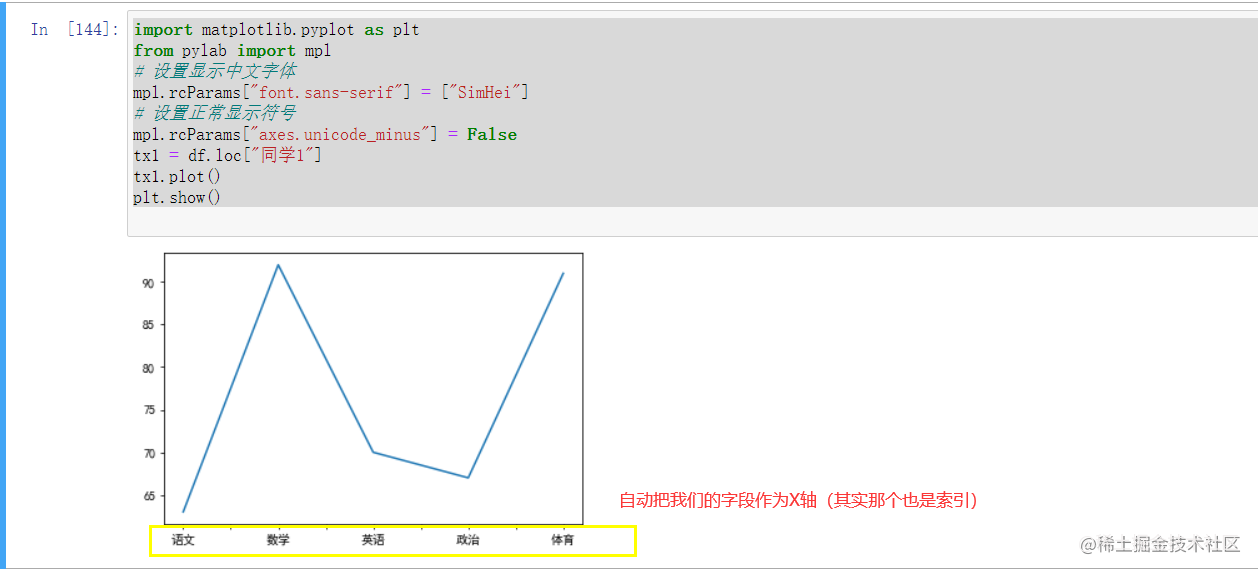DataFrame.plot (kind='line')

line 線

bar 柱狀圖

barh 橫向柱狀圖

scatter 散點圖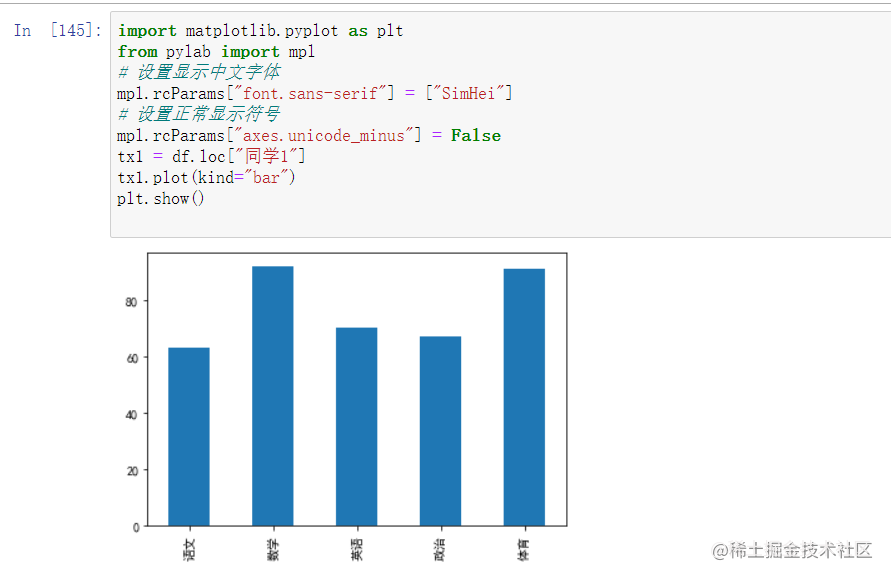## 缺失值處理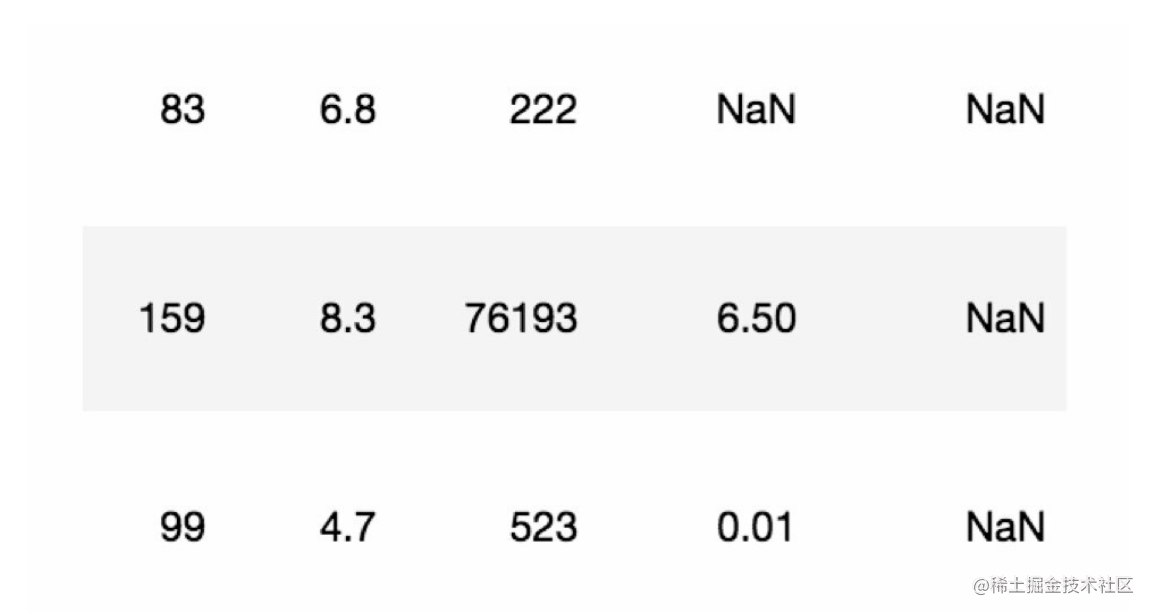1. isnull判斷是否有缺失資料NaN
2. fillna實現缺失值的填充
3. dropna實現缺失值的刪除
4. replace實現資料的替換

5. pd.isnull(df) 為空返回TRUE

6. pd.notnull(df) 不為空返回TRUE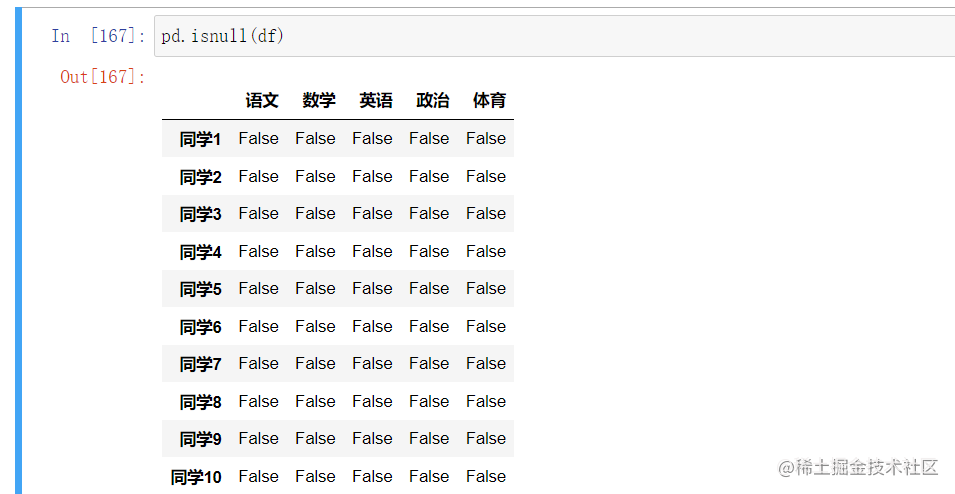```python for i in df.columns: if np.all(pd.notnull(df[i])) == False: print(i) df[i].fillna(df[i].mean(), inplace=True)

# df = df.dropna() 直接刪掉空的

``` 我們使用numpy的all判斷

## 資料離散處理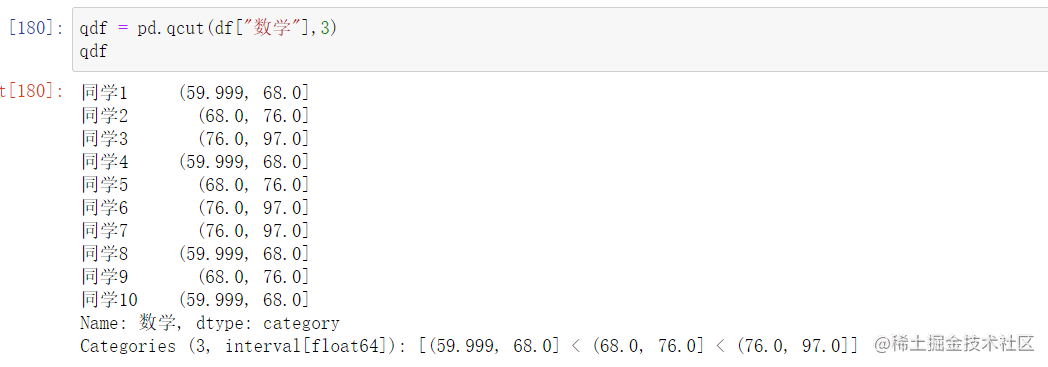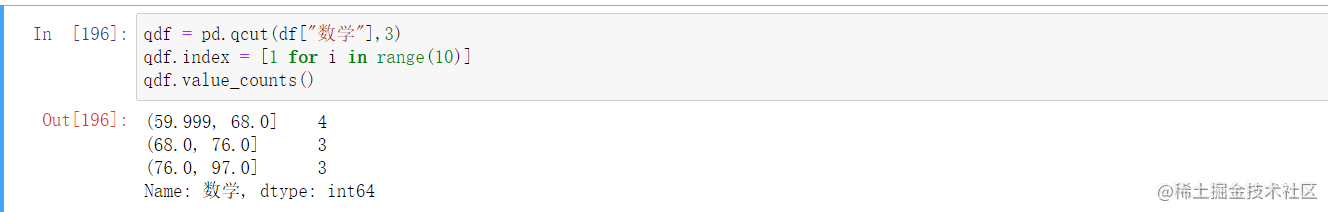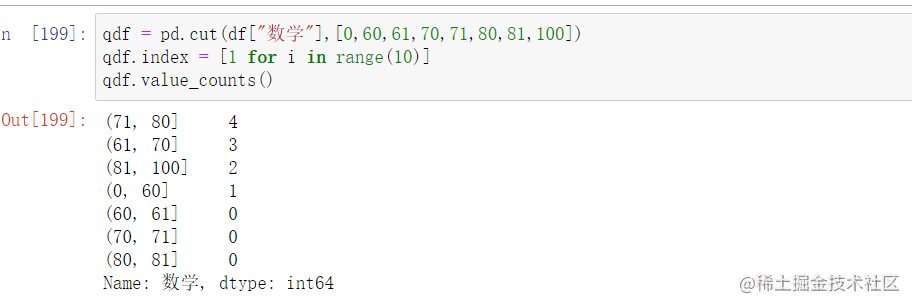## 合併資料

```python import pandas as pd import numpy as np

score = np.random.randint(60, 100, (10, 5)) score2 = np.random.randint(60, 100, (10, 5))

# 構造行索引序列

subjects = ["語文", "數學", "英語", "政治", "體育"]

# 構造列索引序列

stu = ['同學' + str(i) for i in range(1,score.shape+1)]

df = pd.DataFrame(score,columns=subjects,index=stu,dtype=float) df2 = pd.DataFrame(score2,columns=subjects,index=stu,dtype=float) ```

### concat 直接合並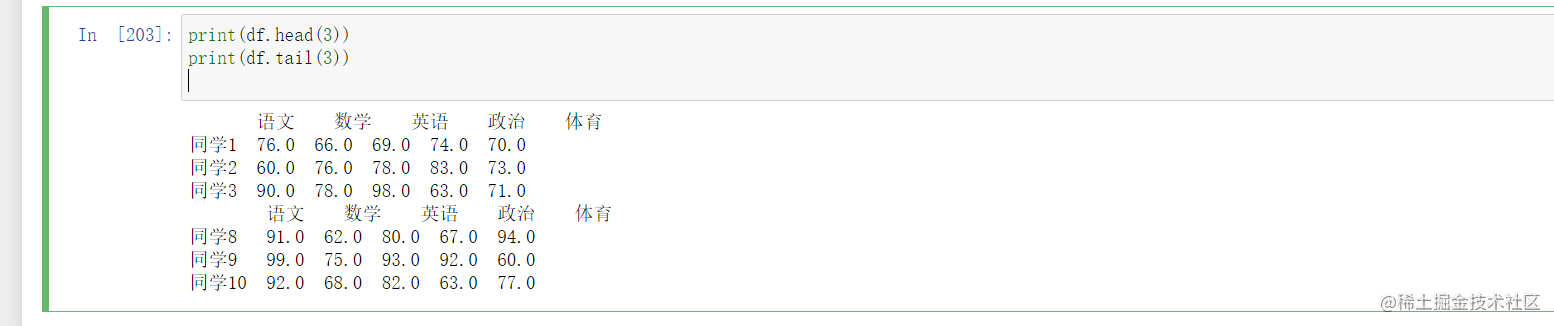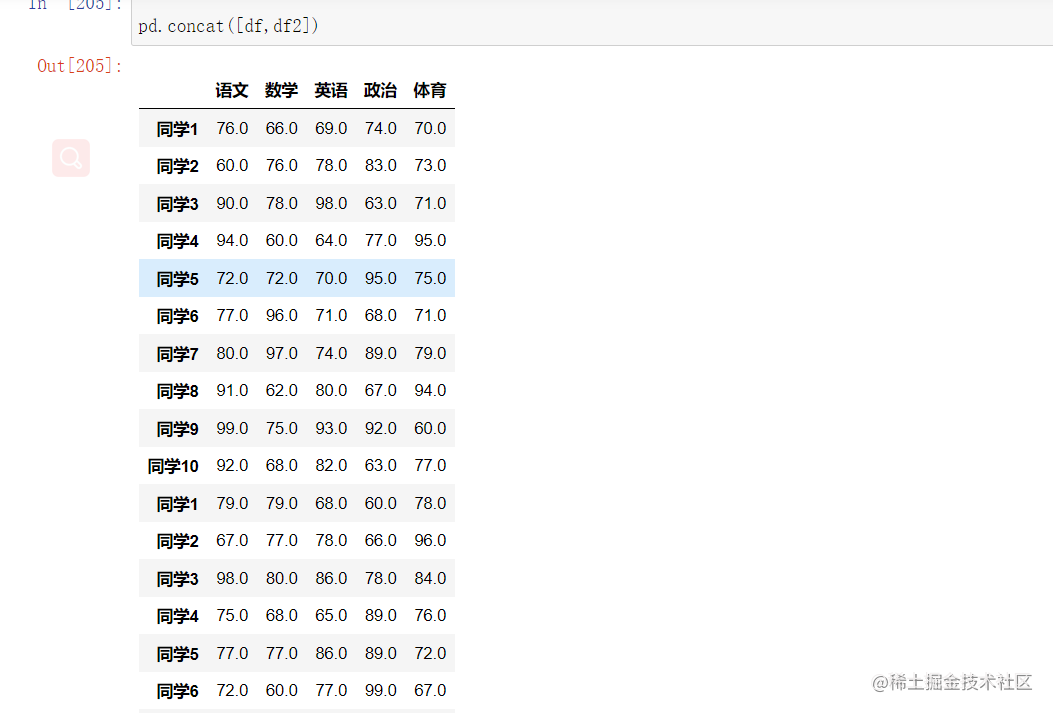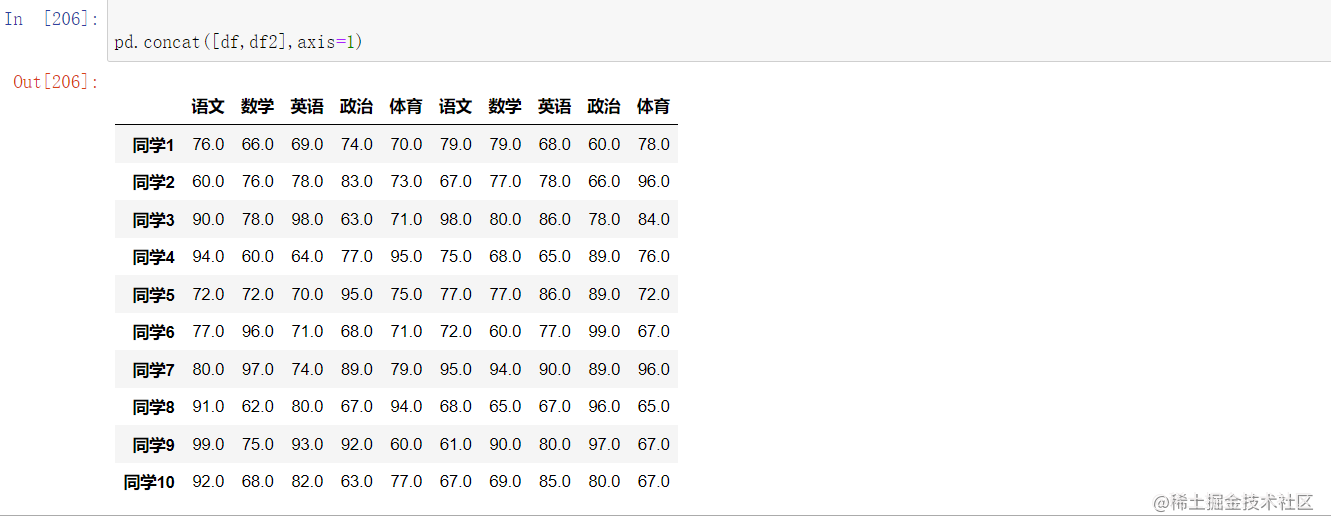### Merge合併

`result = pd.merge(left, right, on=['key1', 'key2'])`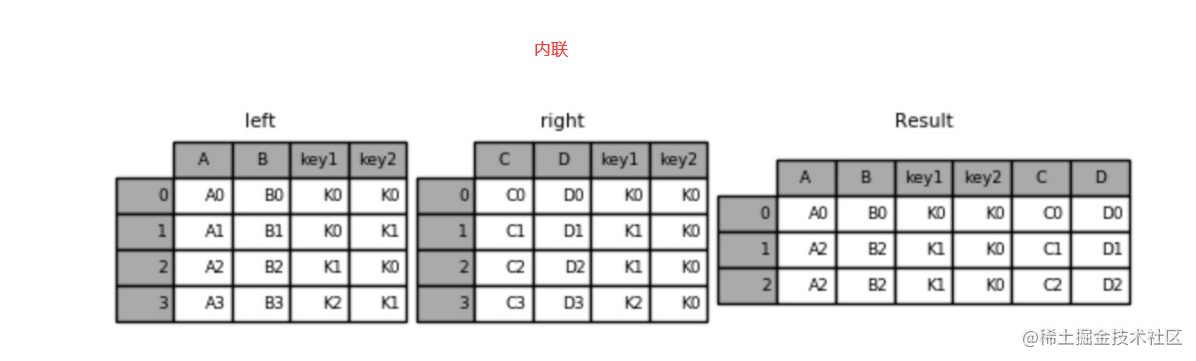`result = pd.merge(left, right,how="left", on=['key1', 'key2'])`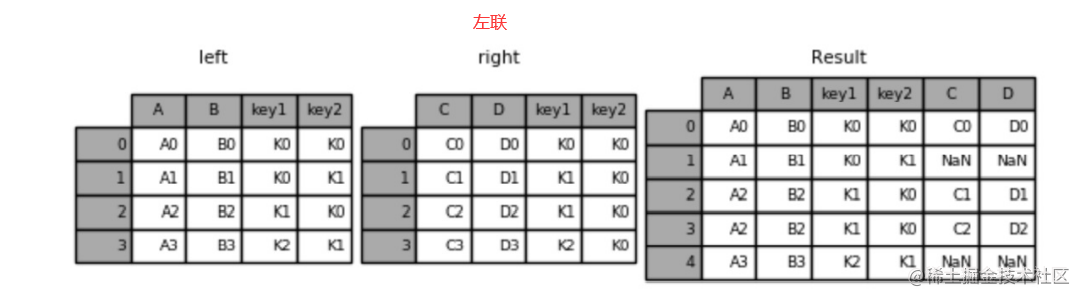`result = pd.merge(left, right,how="right", on=['key1', 'key2'])`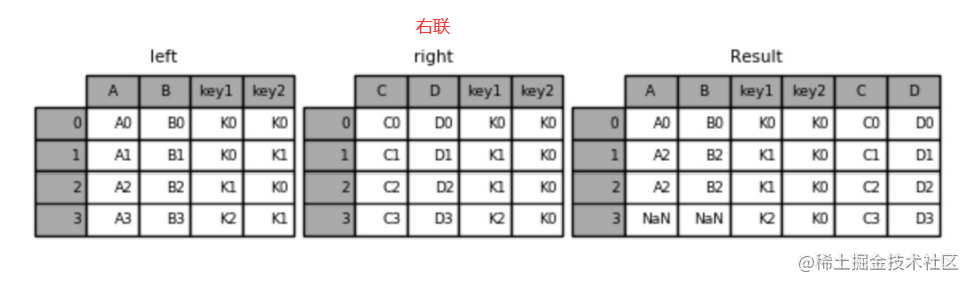`result = pd.merge(left, right,how="outer", on=['key1', 'key2'])`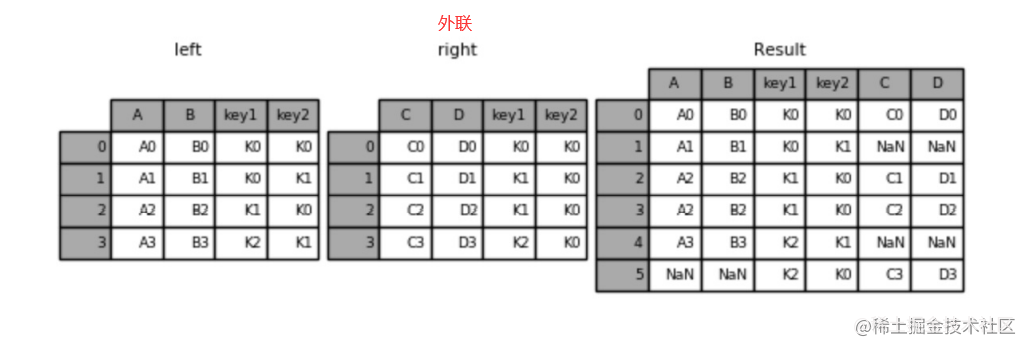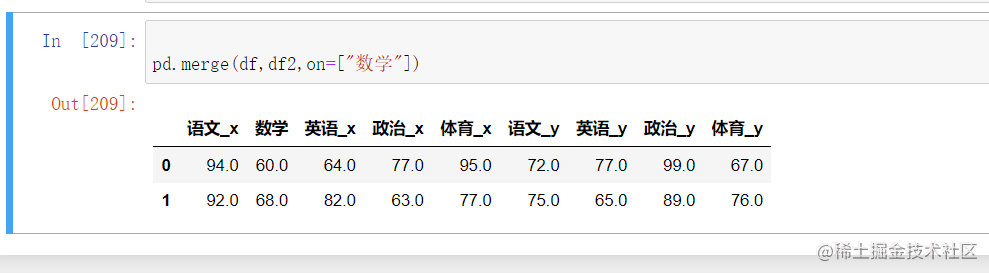## 交叉表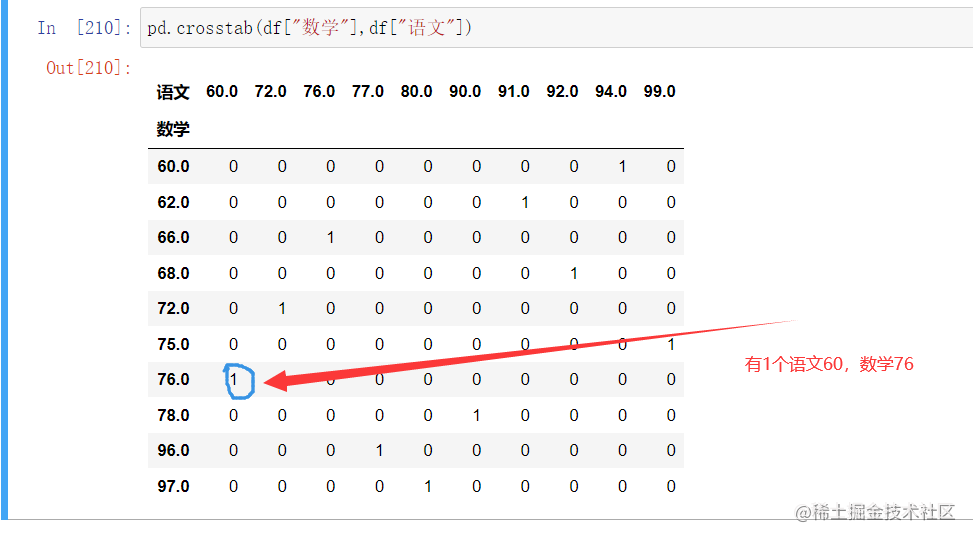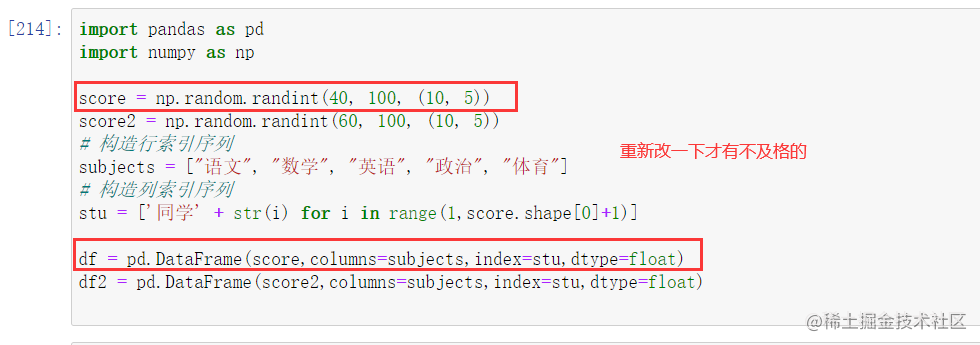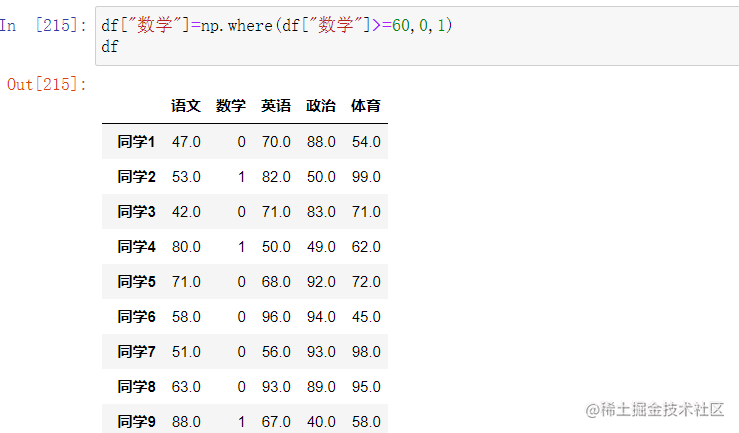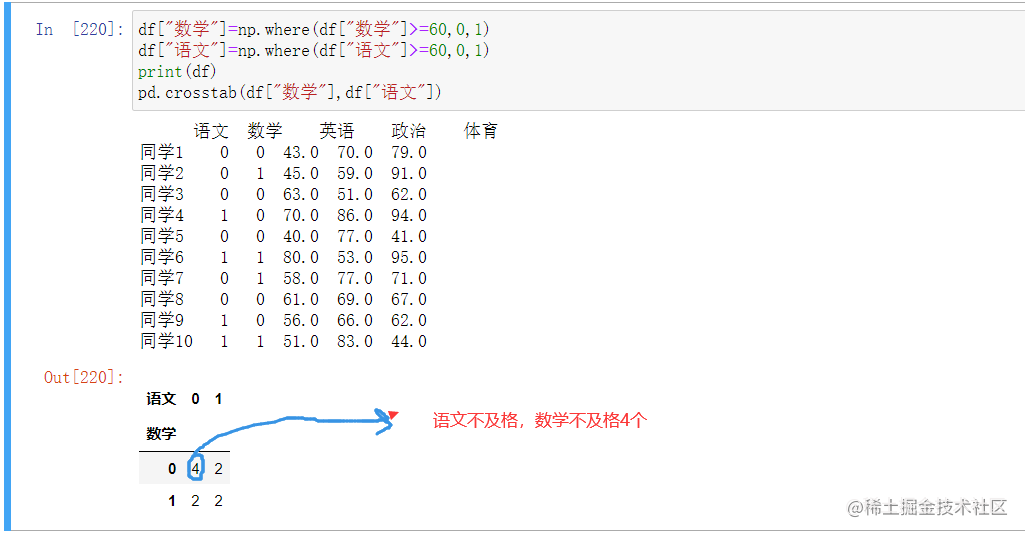```python df["數學"]=np.where(df["數學"]>=60,0,1) df["語文"]=np.where(df["語文"]>=60,0,1)

count = pd.crosstab(df["數學"],df["語文"]) sum = count.sum(axis=1) print(count) pro = count.div(sum,axis=0)

# print(pro)

pro.plot(kind='bar', stacked=True)#TRUE疊加 plt.show()

```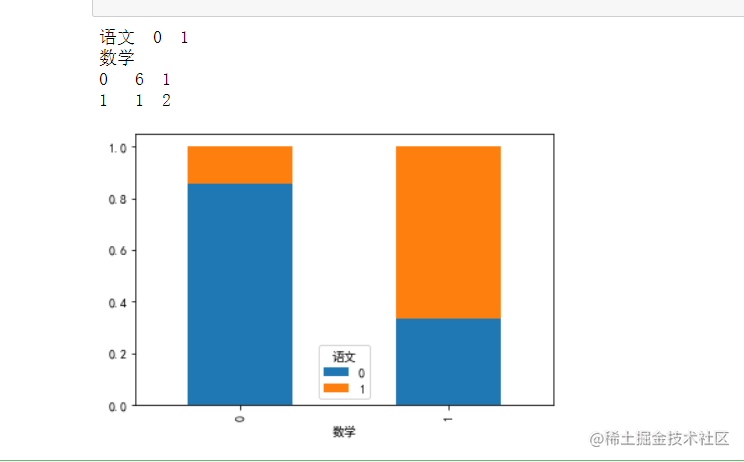## 分組聚合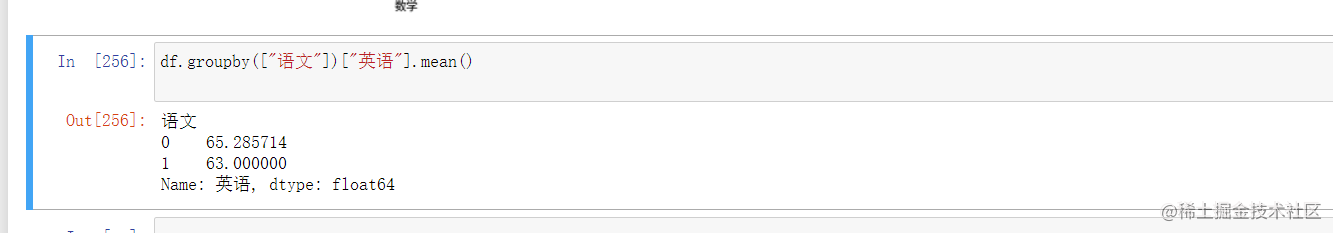# 讀取/儲存檔案

pandas可以讀取多種檔案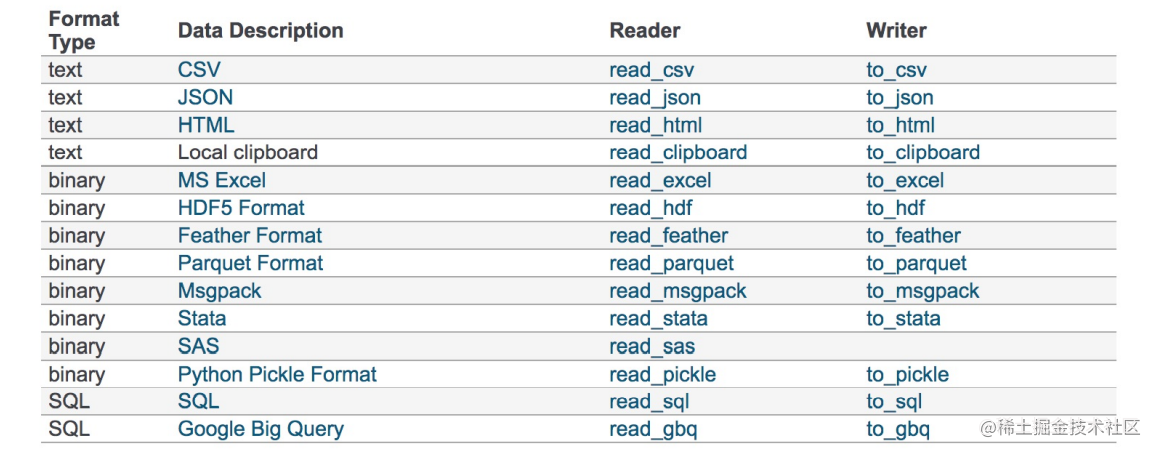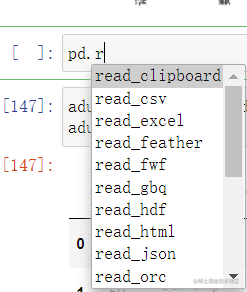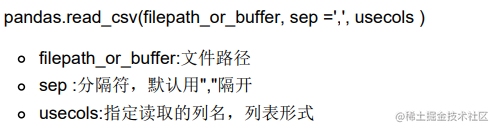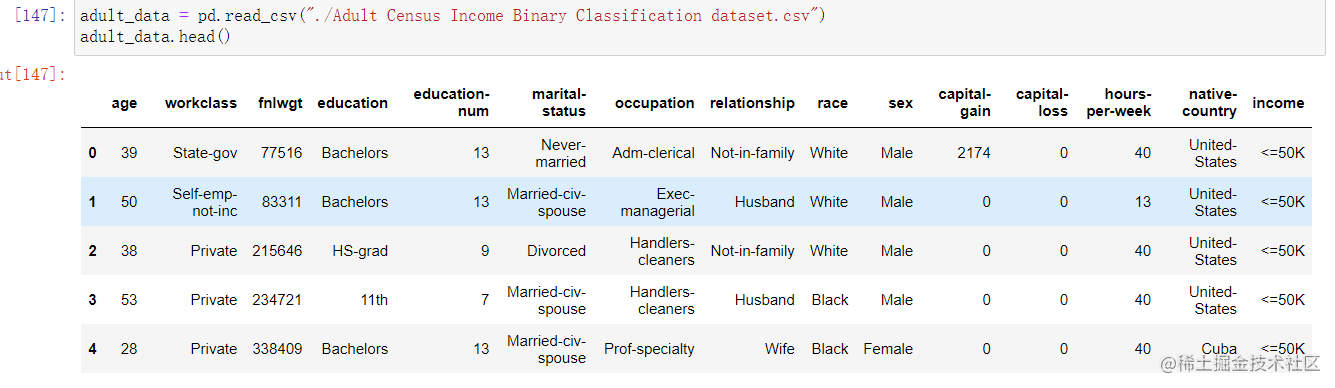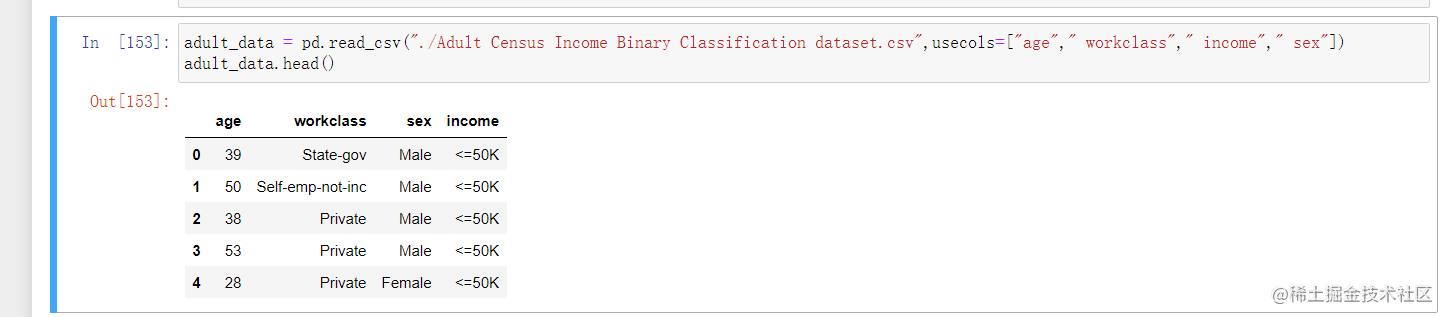之後是寫進去